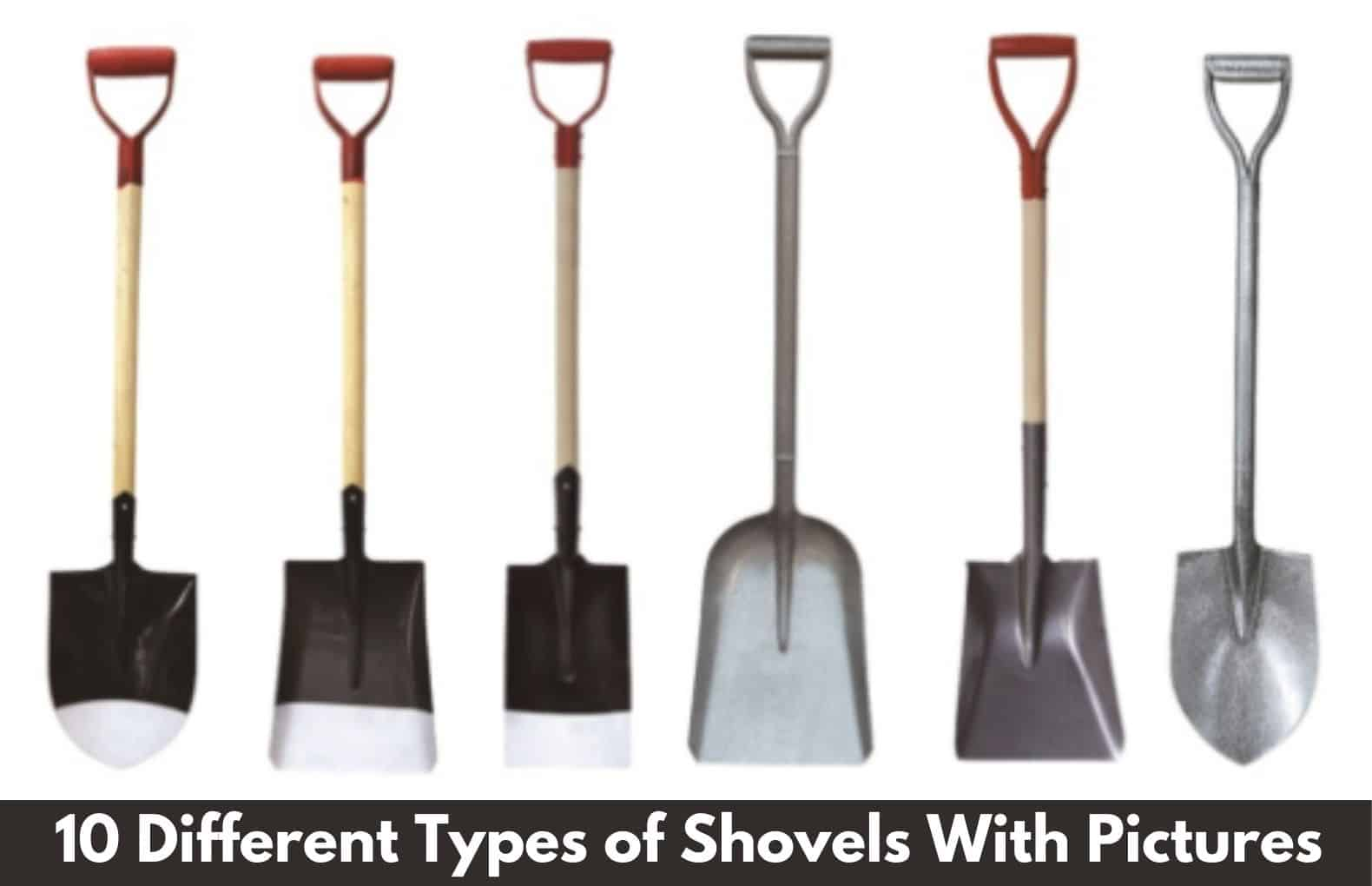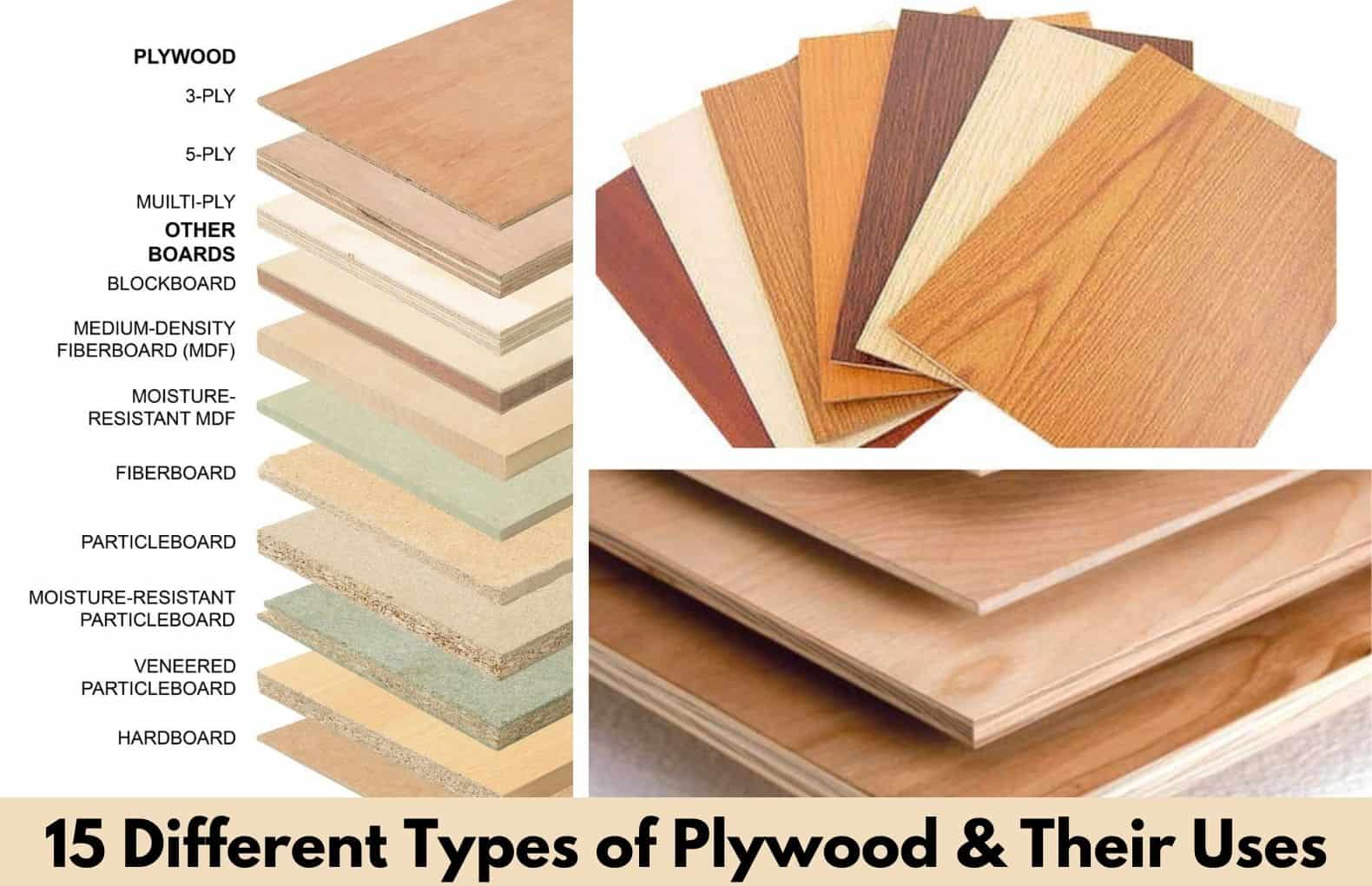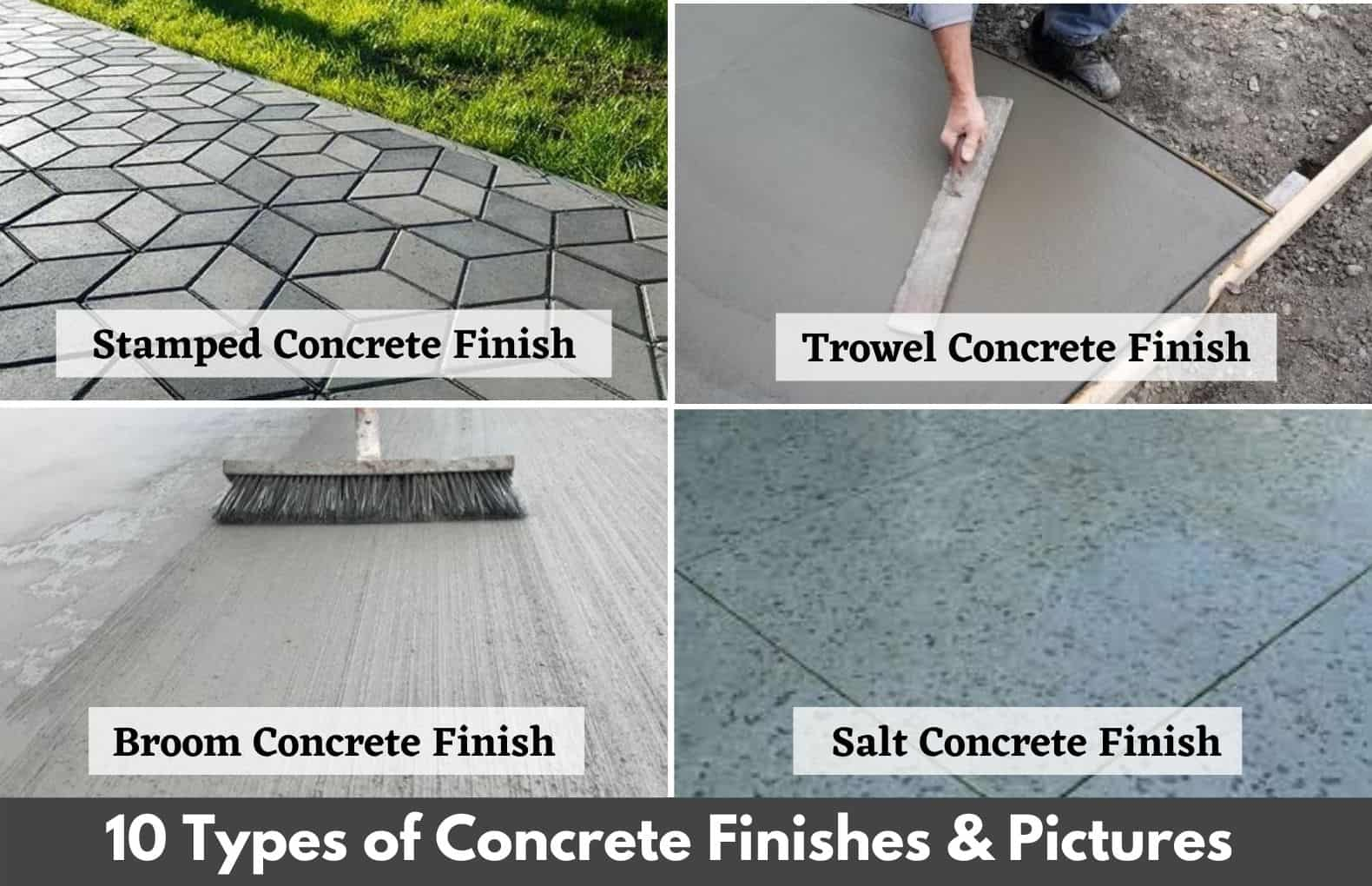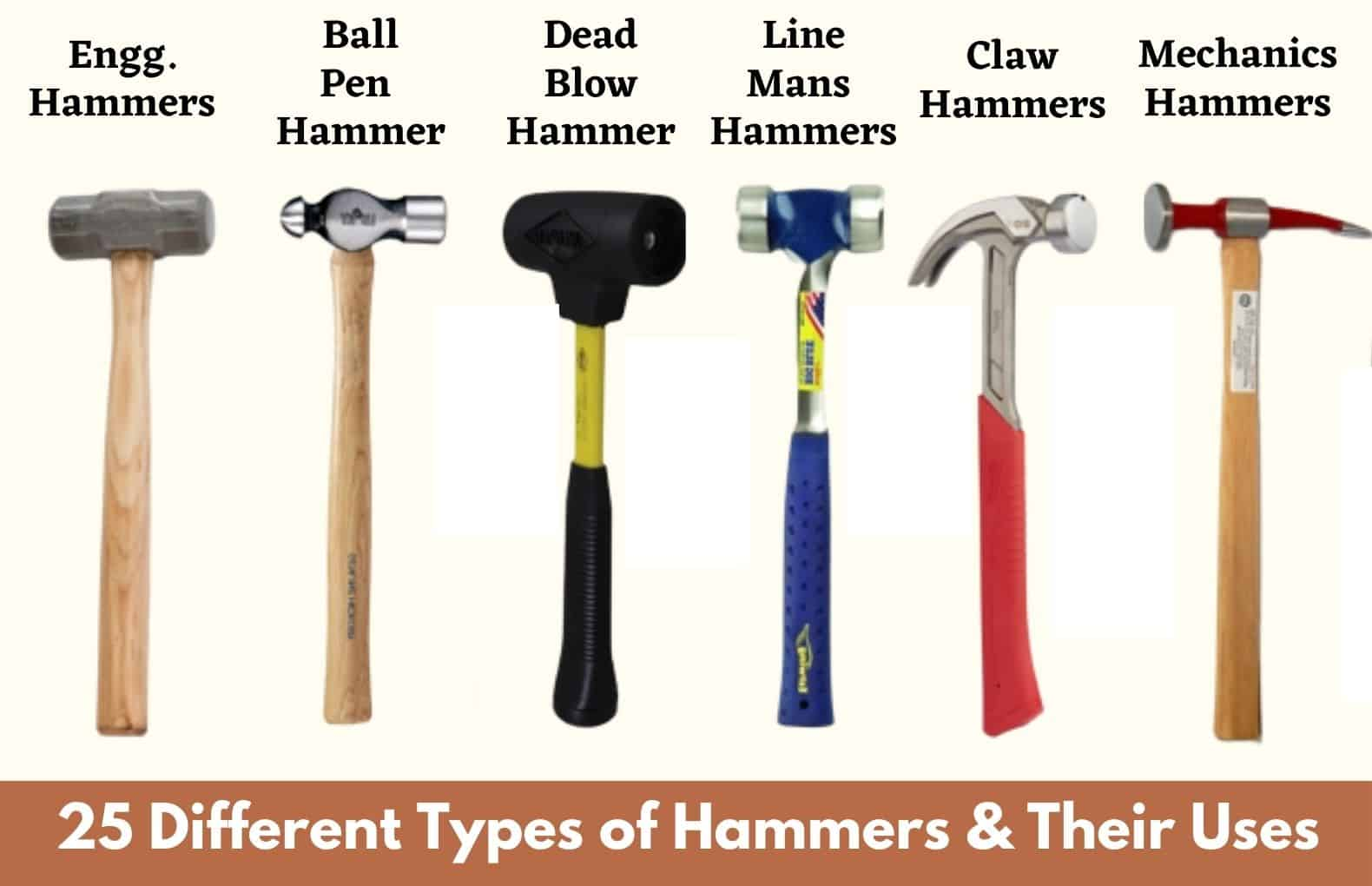# Tile Calculator | Skirting Calculator | How Much Tiles Do I Need | How To Calculate Skirting Tiles | Skirting Tiles Size | How to Measure Floor for Tile11 min read

Post Contents

## Tile Calculator

The following calculator is used to estimate the total number of tiles required to cover a floor, roof, wall, or any other surface.

## What Is Tiles?

tile is thin construction material usually square or rectangular in shape. It is manufactured from a tough  material such as ceramic, stone, metal, baked clay, or even glass, generally used for covering roofs, floors, walls,

## Purpose of Tile Flooring in House

Following are the main purpose of installing tiles in the house,

• Tiles have a polished clean surface, which offers easy cleaning.
• It is easily available in the market and offers various attractive colors, shapes, and designs.
• The tiles are durable construction materials.

The flooring tiles are mostly used in the kitchen, bedroom, leaving room, bathroom, drawing room, terrace, swimming pool and etc.

It is better to choose ISO Certified tiles from a leading brand; I would like to suggest using Lavish Ceramics Floor tiles like:

• Glazed porcelain tiles
• polished glazed vitrified tiles
• double charge tiles
• soluble salt tiles
• wooden tiles
• outdoor tiles

## Standard Size of Flooring Tiles

Floor tiles are generally manufactured in rectangle and square shapes and they come in a variety of sizes to choose from. Some of the standard sizes of floor tiles, wall tiles are given below,

12 inch ×12 inch

18 inch ×18 inch

12 inch ×24 inch

### Wall Tile Size

8 inch×10 inch

10-inch x 12 inch

Bigger than above also used.

Many manufactured even creating the larger size of tiles in sizes of 24”×48”, 36”×36’, and 48”×48”.

• The larger size tiles have minimalist style and design; they are strongly impressive on the floors at first glance. If you like minimalist and modern settings, large floor tiles are perfect for you. And, if you are a fan of classic tile, then there is no limit of choice.
• In the case of kitchen flooring tiles, the 12-inch tiles are standard and are manufactured from different materials like porcelain, ceramic, and various natural stone options.
• The standard size of tile for the bathroom includes 8 inches, 6 inches, 4 inches, and 2 inches. Wall suit for bathroom floor and wall are may be tiny mosaic tiles to gigantic tiles, which can reach meters in length.
• Choosing the right size of floor tile is essential to give your home an elegant appearance.  Selecting a small bathroom floor tile size, you should keep in mind that large tiles create a calm feeling which is perfect for bathrooms with limited space. Select a large size of neutral colors such as white or gray or rectangular-shaped tiles that look fabulous in bathrooms and can be installed vertically and horizontally.

## How many tiles Do I Need? | How Many Tiles Do I Need?

First, we will calculate the number of tiles needed for a floor in an sq. m unit. (Unit of measurement is sq. m.). You can also use the above tile calculator.

### Step- 1 Measure Area of Room or Floor

Take the length and width measurement of your room in the meter unit.

• Suppose, Length of the room floor is 10m and the Width of the room floor is 8m.
• It is a 10m x 8m Room.

### Step – 2 Calculate the Area of the Room or Floor

• The area can be calculated by just multiplying the length and width of the floor together.
• So, Area = Length x Width = 10m x 8m = 80 sq. m.

### Step – 3 Measure the Size of Tiles

• Take measurements of tile selected or propose to be installed in a given flooring area.

Let’s take size of tile is 0.30 m x 0.30 m (30cm x 30cm)

### Step – 4 Calculate the Area of the Tile

• The tile’s area can be calculated by just multiplying its length and width together.
• Area of Tile = 0.60 m x 0.60 m = 0.36 sq. m.

### Step – 5 Calculate the Numbers of Tiles Needed For a Floor

• Nos. of tiles required for a given floor area is calculated by diving the area of floor by area of one tile.
• Nos. of Tiles = Area of Floor / Area of One Tile

= 80 / 0.36 = 222 Nos.

• Note: There are always chances of wastage because sometimes cutting is required in many areas. So, always consider at least 5 % wastage, while calculating the number of tiles needed for a floor.
• Add an Extra 5 % Quantity of tiles for wastage compensation.

Nos. of Tiles = 222 + 5 % Wastage

= 222 + (222 x 0.05)

= 222 + 11 = 233 nos.

## How to Calculate How many tiles I Need for Floor?

Now, we will calculate the number of tiles needed for a floor in sq. ft unit. (Unit of measurement is sq. ft.)

### Step- 1 Measure Area of Room or Floor

• Take the length and width measurement of your room in feet unit.
• Suppose, Length of the room floor is 20 ft. and the Width of the room floor is 15 ft.
• So, it is 20 ft. x 15 ft. Room.

### Step – 2 Calculate the Area of the Room or Floor

• The area can be calculated by just multiplying the length and width of the floor together.
• So, Area = Length x Width = 20 ft. x 15 ft. = 300 sq. ft.

### Step – 3 Measure the Size of Tiles

• Take measurements of tile selected or propose to be installed in a given flooring area.
• Let’s take the size of the tile as 2 ft. x 2 ft. (24-inch x 24 inches)

### Step – 4 Calculate the Area of the Tile

• The tiles’ area can be calculated by just multiplying its length and width together.
• Area of Tile = 2 ft. x 2 ft.=  4 sq. ft.

### Step – 5 Calculate the Numbers of Tiles Needed For a Floor

• Nos. of tiles required for a given floor area is calculated by diving the area of floor by area of one tile.

Nos. of Tiles = Area of Floor / Area of One Tile

= 300 sq. ft. / 4 sq. ft. = 75 Nos.

Note: There are always chances of wastage because sometimes cutting is required in many areas. So, always consider at least 5 % wastage, while calculating the number of tiles needed for a floor.

Add an Extra 5 % Quantity of tiles for wastage compensation.

Nos. of Tiles = 75 + 5 % Wastage

= 75 + (75 x 0.05)

= 75 + 4 = 79 nos.

## How to Calculate Skirting Tiles | How to Calculate Running Feet of Skirting

• Skirting quantity is generally measured in the running meter. So, for calculating the number of skirting tiles required to measure the running meter length of the room.

(Note: skirting is measured in running feet or running meter)

Take the size of the room is 10 m x 8 m.

• Now, Measure the Perimeter or Running meter length of the room.
• If you have the length and width of the room, then you can easily calculate the running meter of the room by just multiplying the length and width twice and summing it.

Running meter length of room = (2 x 10m) + (2 x 8m) = 36m.

• We have to deduct the length of doors because skirting is not provided on the door option.
• Size of Door = 1 m x 2.1m, Length of door = 1 m.
• Actual Running meter length of Skirting = 36 m – 1 m = 35 m.
• So, 36 m is the running meter of room on which skirting tiles are required to install.

## How to Calculate Skirting Tiles For Room

In skirting tiles calculation, a number of skirting tiles can be calculated by just diving the running meter length of the room by the length of the skirting tile selected.

Suppose, skirting tiles size is 30cm x 7cm ( 0.30 m x 0.07 m)

In the above size of tile 0.30m the length of tile and 0.07 m height of the tile.

Number of Skirting Tiles = 35 m / 0.30 m = 116.66 Nos., Correct it = 117 m.

## FAQs:

### Q.1 How do I calculate how many tiles I need?

To calculate the number of tiles required for a floor we should know the area of the floor and the single tile. To do so simply multiply the length and breadth of the floor to know the area and repeat the same for tile. Dividing the area of the floor with tile floor gives us the no. of tiles required for the floor. Round off it to the nearest integer.

### Q.2 How many 12 x 12 (inches) tiles do I need for 100 square feet?

12 x 12-inch tile has an area equal to 1 square foot, i.e 12-inches x 12 inches = 144 sq inches which is equal to 1 sq foot. Hence to accommodate 100 sq feet with tiles we will require 100 such tiles of 12 x 12 inches.

### Q.3 What is the Standard Size of Flooring Tiles?

Tiles for the floor are available in standard sizes of:
12 inch ×12 inch
18 inch ×18 inch
12 inch ×24 inch

### How to Calculate Tiles Needed for a Floor

Multiply the tile length by the width to figure the area that one tile will cover in square inches. Divide the result by 144 to convert it to square feet. Then, divide the area you’re tiling by the square footage of one tile to determine how many tiles you need. Again, round up to the nearest whole number.

### How Many Tiles Come in a Box

8 to 12 Tile per Box
The number of tiles in the box depends on tile size. In 12×12 inch tile, each box contains 8-12 tiles per box.

### Popular Post on Website#### 15 Types of Fans | Different Types of Fans For Home | Ceiling Fan Type | Best Fan Types & Their Uses#### 22 Types of Drill Bits | Drill Bit Types And Uses | Different Drill Bits Name | Best Drill Bits For Cutting Work#### 40 Types Of Tables | Different Types of Tables | Best Types Of Table For Living Room | Different Kinds Of Tables#### 10 Types of Shovels | Different Types of Shovels With Pictures | Best Shovels For Digging Work#### 15 Different Types of Plywood | What Is Plywood and Their Types | 15 Best Types of Plywood for Furniture#### 30 Types of Clamps and Their Uses | 30 Different Types Clamps and Uses | Best Clamps For Home Uses#### 10 Concrete Finishes Types | 10 Best Types of Concrete Finishes | Different Concrete Finishes | Concrete Surface Finishes#### 25 Types of Hammers | Different Types of Hammers and Their Uses | Top 25 Hammers Types#### 10 Different Types of Ladders and Their Uses | Best Types of Ladders | Material Used For Ladders#### 17 Types Of Contractors | What Is Contractor | 17 Different Types of Contractors | List of Contractors#### 13 Types of Door Hinges | Types of Hinges Used For Door | Different Types Of Door Hinges | What Is A Butt Hinge#### 40 Types of Bridges | Classification of Bridges | 40 Different Types of Bridges Used In The World#### 10 Most Popular Types of Fencing | Types of Fence | Different Styles of Fencing | Fences for Yard﻿
x

### Related Posts

What Is Skirting | 12 Types of Skirting | Skirting In Construction | Skirting Meaning | Skirting Wall Design
What Is Skirting? Skirting Tiles Skirting tiles are the Tiles or Board that lay in the corner or junction of the floor and wall. The Skirt...
Setting Time of Cement | Initial and Final Setting Time of Cement Test | Initial Setting Time of Cement | Final Setting Time of Cement
What Is Setting Time of Cement? Initial setting time gives an idea about how fast cement can start losing its plasticity and the final settin...
Which Cement Is Best for House Construction | Which Cement Is Best | Best Cement In India | Best Cement for Slab | Best Cement For Roof
Which Cement Is Best for House Construction Which Cement is Best for House Construction is a common question asked many people who are thinki...